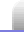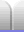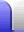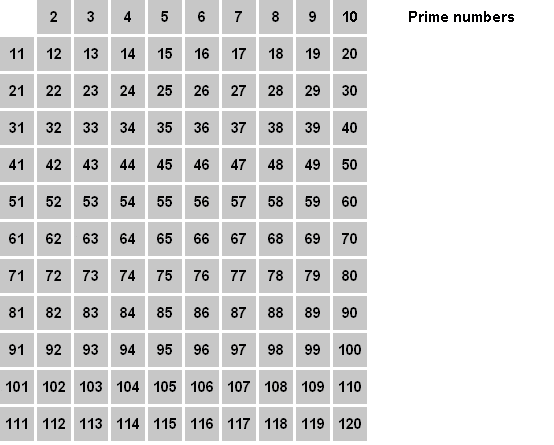# Prime Numbers

### Prime Numbers

A prime number is a number which is only divisible by itself and 1.

The number 1 is not a prime number, so the smallest prime number is 2.

2 is the only even prime number because all other even numbers can be divided by 2.

Prime numbers are essential in modern cryptography.

### Sieve of Eratosthenes

From Wikipedia:

In mathematics, the Sieve of Eratosthenes is a simple, ancient algorithm for finding all prime numbers up to a specified integer. It works efficiently for the smaller primes (below 10 million). It was created by Eratosthenes, an ancient Greek mathematician.The way it works is that when a prime number is found, all multiples of that prime number are eliminated.

The Sieve of Eratosthenes is quite efficient because factors only need to be checked up to the square root of the maximum number in the sieve. (This point is also very useful for factorising numbers in general.) In the above grid, only the numbers 10 and under thus need to be checked, because 112 = 121. Anything that has not been ruled out after these first few factors have been checked is a prime number.

Eratosthenes was a polymath – someone good at a large number of different things. He had many claims to fame, including being the first person to calculate the circumference of Earth. He was only 66 km out (0.16%), which is not bad at all for 240 BC. (Yes, the ancient Greeks not only knew Earth is round, but knew how big it is.)Home Astronomy Chemistry Electronics & Computers Mathematics Physics Field Trips Turn on javascript for email link